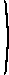# Mathematical methods for economic theory

Martin J. Osborne

## 1.3 Solving systems of linear equations: Cramer's rule and matrix inversion

Consider a system of two equations in two variables x1 and x2:
 ax1 + bx2 = u cx1 + dx2 = v.
One straightforward way to solve for x1 and x2 is to isolate one of the variables in one of the equations and substitute the result into the other equation. For example, from the second equation we have
x2 = (v − cx1)/d.
Substituting this expression for x2 into the first equation yields
ax1 + b(v − cx1)/d = u,
which we can write as
(a − bc/d)x1 + bv/d = u,
so that
x1 =
 u − bv/d a − bc/d
or
x1 =
 ud − bv ad − bc
.
To find x2 we use the fact that x2 = (v − cx1)/d, to get
x2 =
 va − cu ad − bc
.
While this method works well for a system of two equations in two unknowns, its generalization to systems with more equations and variables is cumbersome. A more elegant method that generalizes to any number of equations starts by writing the system in matrix form, asa bc dx1x2
=uv
.     (*)

A general n-equation system may be written as
Ax = b,
where A is an n × n matrix and x and b are n × 1 column vectors. If A is nonsingular, then multiplying each side by the inverse A−1 of A yields
x = A−1b.

If n = 2 or n = 3, the inverse of A is relatively easy to compute, so that the solution of the system can easily be found, as the following examples demonstrate.

Example
Consider the system of equations
 ax1 + bx2 = u cx1 + dx2 = v.
Write it in matrix form. The inverse of the matrix on the left isd −b−c a
so we havex1x2
=d −b−c auv
.
Thus
x1 =
 ud − bv ad − bc
and
x2 =
 va − cu ad − bc
as we found before.
Example
Consider the system of equations
 2 x1 + x2 + 2 x3 = 1 x1 − x2 + x3 = 0 2 x2 − x3 = 3
When written in matrix form, the matrix on the left is2 1 21 −1 1 0 2 −1
.
Using the general formula for the inverse of a matrix, the inverse of this matrix is−1/3 5/3 11/3 −2/3 0 2/3 −4/3 −1
.
Multiplying b, which is the transpose of (1, 0, 3), by this matrix yields (x1x2x3) = (8/3, 1/3, −7/3). (By substituting this list of values into the original equations you can verify that it is indeed a solution—and therefore the solution.)

### Cramer's rule

A useful implication of the fact that the solution of the system Ax = b is given by x = A−1b is the following result (due to Gabriel Cramer, 1704-1752), which gives an explicit expression for the value of each variable separately.
Proposition (Cramer's rule)
Let A be an n × n matrix, let b be a n × 1 column vector, and consider the system of equations
Ax = b.
where x is an n × 1 column vector. If A is nonsingular then the (unique) value of x that satisfies the system is given by
xi = |A*(b,i)|/|A| for i = 1, ..., n,
where A*(b,i) is the matrix obtained from A by replacing the ith column with b.
Proof
We have x = A−1b, so that
xi = ∑n
j=1
vijbj,
where vij is the (i,j)th component of A−1.

Now, by a previous result, the (i,j)th component of A−1 is (−1)i+j|Aji|/|A|. Thus

xi = ∑n
j=1
(−1)i+j|Aji|bj/|A|.
Finally, calculate the determinant of A*(b,i) in the statement of the result by expanding along its ith column. This column is b, so according to the second part of a previous result we have
|A*(b,i)| = ∑n
j=1
(−1)i+jbj|Aji|,
establishing the result. (Note that because i is the index of the column along which we are expanding, the roles of i and j here are reversed relative to their roles in the statement of the result we are using.)
Example
Applying Cramer's rule to the two-variable system
 ax1 + bx2 = u cx1 + dx2 = v
we get
x1 =u bv d
and
x2 =a uc v
.
Calculating the determinants in the numerators, we have
x1 =
 ud − bv ad − bc
and
x2 =
 va − cu ad − bc
.
Cramer's rule is particularly useful if you want to calculate the value of only some of the variables in a solution of a system of equations, as the following example demonstrates.
Example
The value of x2 in a solution of the system of equations
 2 x1 + x2 + 2 x3 = 1 x1 − x2 + x3 = 0 2 x2 − x3 = 3
is, by Cramer's rule, the determinant of2 1 21 0 1 0 3 −1
divided by the determinant of2 1 21 −1 1 0 2 −1
.
The first determinant is 1 and the second is 3, so the value of x2 in the solution of the system is 1/3.